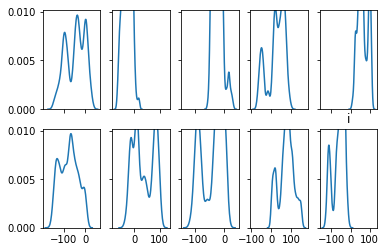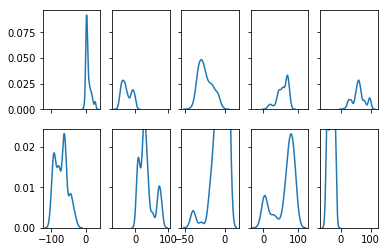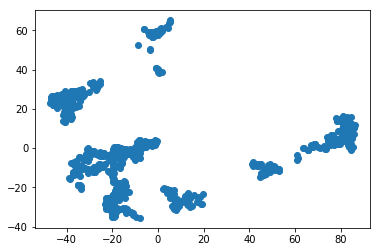(The following is a recreation of the original form of a reponse I submitted on datascience.stackexchange.com prior to significantly editing it. The edited form will correspond to future commits of this notebook.)

TL;DR: Use MCMC to generate samples from p(X|Y) by scoring candidate X values against the class-conditional likelihood provided by your model. MCMC will explore the candidate space of X, but will find and hang around high probability regions and avoid low probability regions.

Here's a concrete demonstration using a random forest classifier:

To start, let's generate a simple multi-class classification problem and train the model:

In :
import numpy as np
from sklearn.ensemble import RandomForestClassifier
from sklearn.datasets import make_blobs

# I didn't actually set a random seed when I originally built this demo,
# so some the text diverges from the results a little in some places. But
# you get the idea.
np.random.seed(123)

X, y = make_blobs(n_samples=1000, n_features=10, centers=5, cluster_std=1.0)

RFC = RandomForestClassifier(n_estimators=80)
RFC.fit(X,y)

Out:
RandomForestClassifier(bootstrap=True, class_weight=None, criterion='gini',
max_depth=None, max_features='auto', max_leaf_nodes=None,
min_impurity_decrease=0.0, min_impurity_split=None,
min_samples_leaf=1, min_samples_split=2,
min_weight_fraction_leaf=0.0, n_estimators=80, n_jobs=1,
oob_score=False, random_state=None, verbose=0,
warm_start=False)

Next, we define our likelihood function by wrapping the model's scoring procedure to spit out an input observation's probability of belonging to the class we're interested in:

In :
def class_cond_prob(x, model=RFC, class_id=0):
if len(x.shape) == 1:
x = x.reshape(1,-1)
return model.predict_proba(x)[0,class_id]


There's probably a way to do this with PyMC3 or something like that, but I don't know my way around that library so I just used a "homebrew" MCMC implementation I found on github (https://gist.github.com/alexsavio/9ecdc1279c9a7d697ed3):

In :
# via https://gist.github.com/alexsavio/9ecdc1279c9a7d697ed3
def metropolis(f, proposal, old):
"""
basic metropolis algorithm, according to the original,
(1953 paper), needs symmetric proposal distribution.
"""
new = proposal(old)
alpha = np.min([f(new)/f(old), 1])
u = np.random.uniform()
# _cnt_ indicates if new sample is used or not.
cnt = 0
if (u < alpha):
old = new
cnt = 1
return old, cnt

def run_chain(chainer, f, proposal, start, n, take=1):
"""
_chainer_ is one of Metropolis, MH, Gibbs ...
_f_ is the unnormalized density function to sample
_proposal_ is the proposal distirbution
_start_ is the initial start of the Markov Chain
_n_ length of the chain
_take_ thinning
"""
count = 0
samples = [start]
for i in range(n):
start, c = chainer(f, proposal, start)
count = count + c
if i%take is 0:
samples.append(start)
return samples, count


Now that we have all the pieces in place, let's generate some samples from p(X|Y=0):

In :
samples, _ = run_chain(chainer=metropolis,
f=class_cond_prob,
proposal=lambda old: old + np.random.randn(1,10),
start=X[y==0,:][0,:],
n=10000,
take=1
)


Let's sanity check our procedure by making sure most of our observations get classified the way we want:

In :
burnin=1000
samples = np.concatenate(samples[burnin:])
y_pred = RFC.predict(samples)
np.mean(y_pred==0) # 0.57

Out:
0.74980557715809359

57% might seem low, but remember: if we were generating random samples we'd expect this proportion to be around 20% (because we have 5 classes), so this is actually pretty reasonable. We could probably improve this a bit by playing with the proposal distribution, thinning, burnin, or maybe even just cranking up the number of samples we draw (but I'm satisfied with this proof-of-concept).

(EDIT: the step size is too big. I tried reducing the proposal variance from 1 to 1/10 and that alone caused the correctly classified proportion to jump to 72%. I'll probably tune this a bit more later, but I didn't want to have to redo all the plots so I'm just adding this note for now)

(EDIT2: Actually, a better solution is to modify the likelihood to collapse the probability of candidates that don't get classified correctly. Will post updated code shortly)

If we want, we could completely constrain our attention to samples that actually get classified the way we want (which I think makes sense in the context of the question):

In :
pos_samples = samples[y_pred==0,:]


Or be even stricter and only keep samples that had really high likelihood scores, say the top 10%:

In :
probs = RFC.predict_proba(pos_samples)[:,0]
top_ix = np.where(probs >= np.percentile(probs, 90))
top_samples = pos_samples[top_ix, :]


Finally, let's visualize the distributions of our respective features, since presumably that really what you're interested in here:

In :
import matplotlib.pyplot as plt
import seaborn as sns

f, axes = plt.subplots(2, 5, sharex='col', sharey='row')
axes = np.concatenate(axes)
for i in range(10):
sns.kdeplot(samples[:,i], ax=axes[i])
plt.title("i")
plt.show()And here're the distributions for just our top 10% of samples

In :
f, axes = plt.subplots(2, 5, sharex='col', sharey='row')
axes = np.concatenate(axes)
for i in range(10):
sns.kdeplot(top_samples[:,i], ax=axes[i])
plt.show()Now, be careful how you use this information. Keep in mind: these are marginal densities, so they aren't giving us information about potentially important interactions. Because we generated the training data, we know that this class is actually a spherical (gaussian) cluster, but the random forest doesn't know that and may not be representing p(X|Y) so cleanly. We should suspect that this is the case because of the appearance of multimodal distributions in those feature distribution plots. If we visualize the 2D PCA projection of our top samples, we can see that the random forest identified several distinct high probability regions for this class, although we know apriori that there should really just be one (at the mean of the gaussian):

In :
from sklearn.decomposition import PCA

pca = PCA(n_components=2)
X_r = pca.fit_transform(top_samples)

plt.scatter(X_r[:,0], X_r[:,1])
plt.show()